Negative Value Under the Square Root Radical MathBitsNotebook.com Terms of Use   Contact Person: Donna RobertsLet's investigate what happens when negative values appear under the radical symbol (as the radicand) for cube roots and square roots.

In some situations, negative numbers under a radical symbol are OK. For example,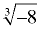is not a problem since (-2) • (-2) • (-2) = -8, making the answer -2. In cube root problems, it is possible to multiply a negative value times itself three times and get a negative answer.

Difficulties, however, develop when we look at a problem such as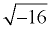. This square root problem is asking for a number multiplied times itself that will give a product (answer) of -16. There simply is no way to multiply a number times itself and get a negative result. Consider: (4) • (4) = 16 and (-4) • (-4) = 16.

 CUBE ROOTS: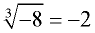BUT SQUARE ROOTS: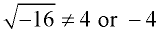Yes, (-2) x (-2) x (-2) = -8. No problem. Nope!   (4) x (4) ≠ -16. Nope!   (-4) x (-4) ≠ -16.Square roots are the culprits! The difficulties arise when you encounter a negative value under a square root. It is not possible to square a value (multiply it times itself) and arrive at a negative value. So, what do we do?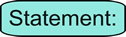The square root of a negative number does not exist among the set of Real Numbers.

When problems with negatives under a square root first appeared, mathematicians thought that a solution did not exist. They saw equations such as x2 + 1 = 0, and wondered what the solution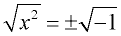really meant.

In an effort to address this problem, mathematicians "created" a new number,
i, which was referred to as an "imaginary number", since it was not in the set of "Real Numbers". This new number was viewed with much skepticism. The imaginary number first appeared in print in the year 1545.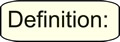The imaginary number "i" is the square root of negative one.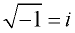An imaginary number possesses the unique property that when squared, the result is negative.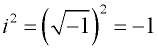Consider: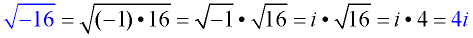The process of simplifying a radical containing a negative factor is the same as normal radical simplification. The only difference is that the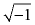will be replaced with an "i ".

As research with imaginary numbers continued, it was discovered that they actually filled a gap in mathematics and served a useful purpose. Imaginary numbers are essential to the study of sciences such as electricity, quantum mechanics, vibration analysis, and cartography.

When the imaginary i was combined with the set of Real Numbers, the all encompassing set of Complex Numbers was formed.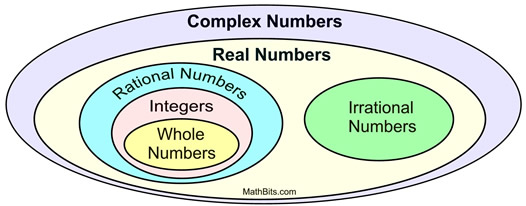Product Rule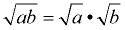where a ≥ 0, b≥ 0 "The square root of a product is equal to the product of the square roots of each factor." This theorem allows us to use our method of simplifying radicals.

 Imaginary (Unit) Number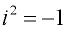Product Rule (extended)where a ≥ 0, b≥ 0 OR a ≥ 0, b < 0 but NOT a < 0, b < 0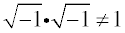FYI: The powers of i always cycle through only 4 different values: i1 = i i2 = -1 i3 = -i i4 = 1 i5 = i and the cycle starts again.

 When doing arithmetic on i, treat it as you would an "x". 3i + 4i = 7i 2i • 4i = 8i2 = -8 Note: i2 was replaced with -1.

 Do NOT confuse "irrational" numbers with "imaginary" numbers. They are NOT the same.

 Complex Numbers a ± bi where a and b are real numbers, and i is the imaginary unit.A complex number is a real number a, or a pure imaginary number bi, or the sum of both.
Complex numbers are written in the standard form a + bi.

In Algebra 1, we will only take a glimpse at "imaginary" numbers. We will see them briefly referenced again under "Quadratics" on the page "Quadratic Equations with Imaginary Roots".
You will study about imaginary numbers in Algebra 2.